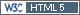## expsampleN.TOST vs. sampleN.TOST [R for BE/BA]

Hello to everyone! I would like to get information about difference between functions expsampleN.TOST and sampleN.TOST. I've read topics at this forum which were contained some data about function expsampleN.TOST. As I noticed expsampleN.TOST is used when a pilot study was conducted and we are able to input the data from that study (estimated CV, m or df, design) to launch the function and to get a result.

So I have 4 questions:
1. The fundamental difference between expsampleN.TOST and sampleN.TOST?
2. CV in function expsampleN.TOST is CVintra, isn't it?
3. In both cases we can choose the value of desired power (for example 0,8 or 0,9). But in description of function expsampleN.TOST the power is characterized as "expected" (sample size based on expected power) and in description of function sampleN.TOST the power is characterized as "given" (calculates the necessary sample size to have at least a given power). What is the difference between expected and given power?
4. Can I use expsampleN.TOST when I did not conduct a pilot study but I have data from other BE studies, information about which was published? If yes, function expsampleN.TOST seems to have advantages over function sampleN.TOST because in a former case I can take into account uncertainty of CV (as we know CVintra is characterized as a parameter with some degree of uncertainty in general sence).Ing. Helmut Schütz